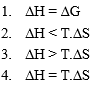Consider the reaction

N2(g) + 3H2(g)$\to$2NH3(g) carried out at constant temperature and pressure. If $∆$H and $∆$E are enthalpy change and internal energy change respectively, which of the following expression is True?

Concept Questions :-

Enthalpy and It's Type
High Yielding Test Series + Question Bank - NEET 2020

Difficulty Level:

Which work done is maximum?

1. Isobaric work           2. Isothermal work

3. Isochoric work         4. Adiabatic work

Concept Questions :-

Thermodynamics' Properties and process

Difficulty Level:

For exothermic reaction to be spontaneous ($∆\mathrm{S}$=negative)

1. Temperature must be high

2. Temperature must be zero

3. Temperature may have any magnitude

4. Temperature must be low

Concept Questions :-

Spontaneity and Entropy
High Yielding Test Series + Question Bank - NEET 2020

Difficulty Level:

Which of the following conditions should be satisfied for the given reaction to be spontaneous at 0$°$C and 1 atm?

H2O(s) $⇌$H2O(l)Concept Questions :-

Spontaneity and Entropy
High Yielding Test Series + Question Bank - NEET 2020

Difficulty Level:

'The free energy change due to a reaction is zero when

1. The reactants are initially mixed.

3. The system is at equilibrium

4. The reactants are completely consumed

Concept Questions :-

Gibbs Energy Change

Difficulty Level:

An ideal gas expands at a constant external pressure of 2.0 atmosphere by 20 litre and absorbs 10kJ of heat from surrounding. What is the change in internal energy of the system:-

(1) 4052 J

(2) 5948 J

(3) 14052 J

(4) 9940 J

Concept Questions :-

First Law of Thermodynamics
High Yielding Test Series + Question Bank - NEET 2020

Difficulty Level:

The temperature of an ideal gas expansion increases in an:-

(2) Isothermal expansion

(4) Isothermal compression

Concept Questions :-

Thermodynamics' Properties and process
High Yielding Test Series + Question Bank - NEET 2020

Difficulty Level:

36 ml of pure water takes 100 sec to evaporate from a vessel and heater connected to an electric source which delivers 806 watt. The $∆{\mathrm{H}}_{\mathrm{vaporisation}}$ of H2O is:-

(1) 40.3 kJ.mol

(2) 43.2 kJ/mol

(3) 4.03 kJ/mol

(4) None of these

Concept Questions :-

First Law of Thermodynamics
High Yielding Test Series + Question Bank - NEET 2020

Difficulty Level:

5 moles of an ideal gas expands isothermally and irreversibly from a pressure of 10 atm to 1 atm against a constant external pressure of 1 atm. find the Wirr at 300 K:-

(1) -15.921 kJ

(2) -11.224 kJ

(3) -110.83 kJ

(4) None of these

Concept Questions :-

Internal Energy and work done
High Yielding Test Series + Question Bank - NEET 2020

Difficulty Level:

In the reaction at 300 K

H2(g) + Cl2(g)$\to$2HCl(g)       $∆\mathrm{H}°$= -185 kJ

if 2 mol of H2 completely react with 2 mole of Cl2 to form HCl. What is $∆\mathrm{U}°$ for this reaction:-

(1) 0

(2) -185 kJ

(3) -370 kJ

(4) None of these

Concept Questions :-

Enthalpy and It's Type